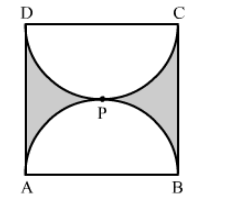# Find the perimeter of the shaded region in the figure,`
Question:

Find the perimeter of the shaded region in the figure, if ABCD is a square of side 14 cm and APB and CPD are semicircles.Solution:

Permieter of shaded region = Length of the arc APB + Length of the arc CPD + Length of AD + Length of BC

$=\frac{1}{2} \times 2 \pi r+\frac{1}{2} \times 2 \pi r+14+14$

$=2 \pi r+28$

$=2 \times \frac{22}{7} \times \frac{14}{2}+28$

$=72 \mathrm{~cm}$

Hence, the perimeter of the shaded region is 72 cm.